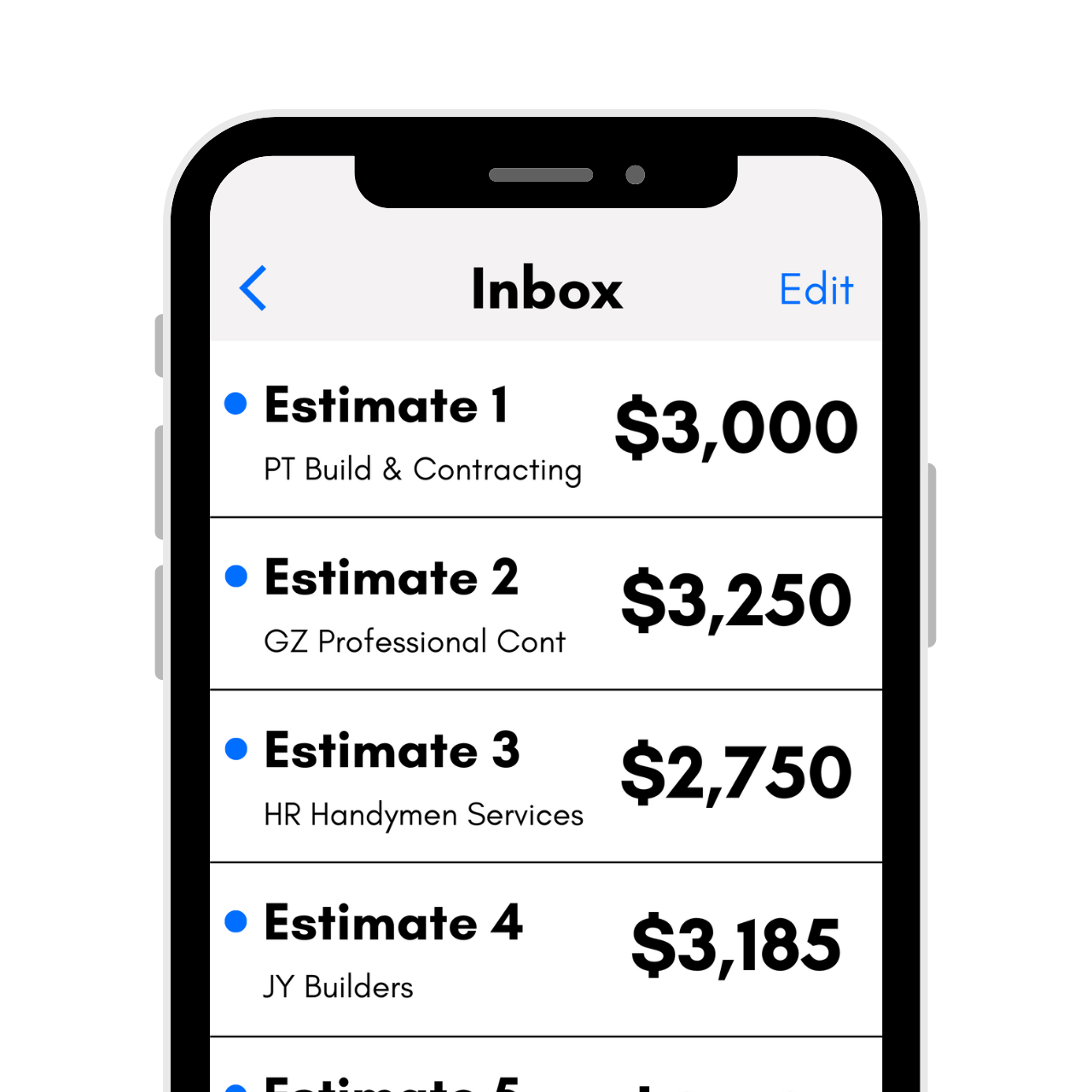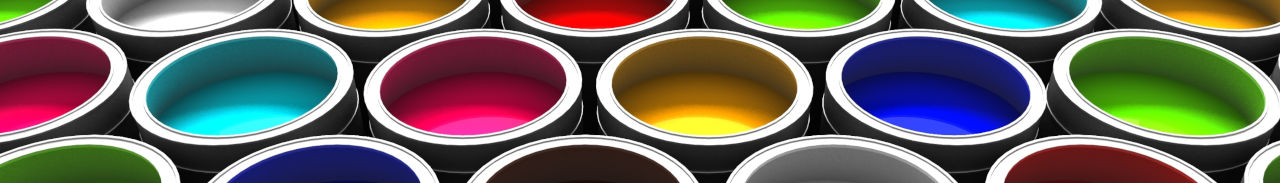# Paint Calculator

Calculate how much paint and primer you need by entering the dimensions of your room below. Optionally, include ceiling paint in your estimate.

Room Dimensions

## Paint Estimate:

gallons of wall paint

gallons of ceiling paint

gallons of primer
Paint Area

wall square feet

ceiling square feet

total square feet
Learn how we calculated this below

## How to Calculate Paint and Primer

When you’re painting a room, a fence, or your whole house, you might be wondering how many gallons of paint you’ll actually need. There are a few methods to figure this out.

The first method is to use the paint calculator above. It estimates the amount of paint, primer, and ceiling paint you’ll need for any project.

Simply enter the size of the area, and the calculator finds the square footage of the walls, then estimates the paint and primer needed for that area.

Of course, you can estimate paint without a calculator as well. You can calculate how many gallons you need in a few simple steps.

Get free estimates from painting pros in your area to compare prices## How Many Gallons of Paint Do You Need?The first step is to calculate the size of the area you will be painting. Paint is a most costly material, and while you do want a small amount extra for touch-ups later, you don’t want to order much more than you need, as it is not returnable.

By far, the easiest way to estimate how many gallons of paint you need for a project is to consult the quick reference table below.

### Paint Coverage Estimates

This table shows the estimated amount of paint needed for average-sized rooms.

One gallon of typical latex paint usually covers 350 to 400 square feet of wall area.

This does assume that the paint you are using can cover in one coat. If you are going from light to dark or dark to light, you may need either a tinted primer and one to two coats of paint or two to three coats of paint without primer to get the desired color.

Paints that contain paint and primer in one will usually cover in one coat, but always check the brand and type of paint you’re using for recommendations, particularly for bright or dark colors. If you’re painting new drywall, then it’s a good idea to apply a coat of primer first.

Amount of paint needed to cover the average-sized room, using a standard latex paint
Room Size Gallons of Paint Needed
small room (8′ x 8′) 1 gallon
medium room (12′ x 12′) 2 gallons
large room (18′ x 18′) 3 gallons
bathroom 1 gallon
kitchen 1-2 gallons
bedroom 2 gallons
family room 3 gallons

### How to Measure a Room

Using a reference table is quick and easy, but the most accurate method of calculating paint and primer for a project is to measure the surfaces to be painted and use the coverage estimate for the specific product you’re using.

#### Step One: Measure the Walls

The first step in estimating paint material is to measure the walls or ceilings you will be covering. Measure the length and height of each wall in feet and write them down. It may be easiest to round up to the next foot measurement for measurements that contain partial feet.

You can also measure the walls in inches and convert the area to square feet later for more accurate measurements, particularly in small spaces, closets, or small bump-out walls.

#### Step Two: Calculate Square Footage of Each Surface

The next step in the process is to calculate the square footage of the walls and ceilings. Square footage is an area measurement of a space, measured in feet, and we have a simple calculator to help with these calculations.

For each set of measurements from step one, multiply the width and height together to get the square footage.

If you measure in inches, divide the number you get from multiplying by 144 to convert to square feet. If doing this method, it is sometimes easiest to add up the square inches from each area first, then convert to square feet. Write down the square footage of each wall.

For example, let’s find the square footage of a wall that is 10 ft. wide by 9 ft. high.

sq ft = 10 ft. × 9 ft.
sq ft = 90 sq ft

Pro Tip: use an area calculator to find the area of complicated wall shapes.

For walls that have large banks of doors or windows, consider subtracting the area of those from the wall square footage to avoid ordering too much paint. To do that, measure the length and height of each door and window and multiply to find the square footage. Then, subtract the door and window square footage from the wall square footage to find the area that needs paint.

If the walls are not simple rectangles or are complex, then consider breaking the wall into smaller sections and calculate the square footage of each section individually. To illustrate this, if your wall is not a normal rectangle, break it into different sections and measure the square footage of each section. Learn more about how to measure irregular walls.

#### Step Three: Add Square Footages Together to Find the Total

Once you know the square footage of each wall, add them together to find the total square footage. If you had split complex walls into several surfaces, then be sure to add each surface to get the total.

For example, let’s find the total square footage for two walls that are 120 square feet and two walls that are 150 square feet.

total sq ft = 120 + 120 + 150 + 150
total sq ft = 540 sq ft

#### Step Four: Calculate Paint Coverage

The final step is to find the coverage ratio of your paint and calculate the number of gallons needed to cover the total square footage. Most professionals use the formula of 350 to 400 square feet of wall coverage per gallon of paint and 200 square feet of wall coverage per gallon of primer.

It’s important to note that different types and manufacturers may cover more or less area. It’s also important to note that different application methods, such as spraying, rolling, or brushing, will cover differently.

These figures also assume that you will be giving an even, solid coat of paint. If you are choosing to use a faux finish, this will change the calculations as well.

Your paint vendor should be able to tell you the coverage for your selected paint, and it’s often indicated on the container.

Divide the total square footage by the coverage rate to find the number of gallons needed.

For example, let’s find the paint needed to cover 540 square feet using a product that covers 350 square feet per gallon.

gallons = sq ft ÷ coverage
gallons = 540 ÷ 350
gallons = 1.54 gallons

You’ll need to round up to the nearest full gallon or quart. It is not a bad idea to have some leftover paint at the end of a project since it can be used for touch-ups, particularly with some shades that can be difficult to match or that can vary slightly from lot to lot.

## How to Estimate Paint for Trim

Here’s a tip: estimate the paint and primer needed to cover trim or small surfaces by taking the linear footage of the room – or the number of feet around the perimeter, and multiplying this by the height of the trim. To make it more accurate, measure the perimeter in inches or divide the linear feet by 12 before multiplying.

Once you have the total square inches, divide by 144 to get the square feet. This can be an easier method than attempting to find the height in feet of some trims, like a pencil, which may be less than 1 inch tall.

You can also estimate trim using the measurement method above. Start by measuring the height of the trim in inches, then convert that to feet. For example, trim that is 4 inches tall is 0.33 feet tall.

Then multiply the height by the length of trim to find the square footage. Then follow the rest of the steps above to estimate the paint needed to cover that square footage.

## How to Estimate Exterior Paint

You can estimate the exterior paint you’ll need just like interior projects. Start by measuring each exterior wall of the home, then calculate the square footage of each one.

For walls with a gable, measure the square portion and the gable portion separately. You might find a triangle area calculator helpful to find the square footage of the gable.

Then, add the square footage of each area together. Subtract the square footage of any doors and windows that you won’t be painting from the total square footage.

Consult the directions on the specific product you’ll be using for a coverage estimate.

Keep in mind that walls with porous surfaces such as concrete, brick, or rough wood will soak up much more paint than a smooth surface. We suggest ordering more paint than you estimate to cover these surfaces.

Or speak to the paint retailer about what they recommend for painting these surfaces. Likewise, a home with wood siding that is older will require more paint than a home with new wood siding.

When making dramatic color changes, you might also need an additional coat of paint to get adequate coverage; be sure to account for this in your estimate.

You can also use our fence stain and deck stain calculators to estimate how much you’ll need.

## Estimation Tips

It may be a good idea to order 10-20% extra, probably an extra quart or gallon, depending on your project, to allow for differences in coverage and to have extra material for touch-ups down the road. If you are fairly close to an even gallon on a small project and want to save some money, consider ordering a quart for your overage.

Don’t forget to estimate other supplies needed for a painting project. Learn more about the costs associated with a painting project.

Be sure to grab plenty of tarps and use a good blue masking tape to tape off any surfaces for a clean paint job. Finally, make sure you choose a good brush and rollers; spending an extra dollar or two on these will have just as much impact on your finish as the quality of the paint itself.

You might also be interested in estimating wallpaper for your space.